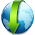Tuesday, January 28, 2014

How to do integration in MATLAB?

MATLAB 2013.b supports direct integration.
It supports both definite and indefinite integrals.
However, if complicated functions are given you will see a message in the command window stating that the explicit integral could not be found. For definite integrals, the approximate result can be obtained by using 'double' function for the result of integration. If the integration results in a singular result, this won't work.

Format:
syms x;
y=int(sin(x),x);

The first line is for declaring 'x' as a symbolic variable.
This will result in
y=-cos(x)

If you are using definite integral,if you enter double(y), it will return the approximate value of the integration.
Try some examples on your own.

1.2.Hi,
I found a 74% OFF coupon to Learn Matlab Udemy course
Couponcode: MATLAB25
https://www.udemy.com/learn-matlab/?couponCode=MATLAB25
3.4.